×#### Thank you for registering.

One of our academic counsellors will contact you within 1 working day.

Click to Chat

1800-1023-196

+91-120-4616500

CART 0

• 0

MY CART (5)

Use Coupon: CART20 and get 20% off on all online Study Material

ITEM
DETAILS
MRP
DISCOUNT
FINAL PRICE
Total Price: Rs.

There are no items in this cart.
Continue Shopping• Complete JEE Main/Advanced Course and Test Series
• OFFERED PRICE: Rs. 15,900
• View Details

```Chapter 10: Direct and Inverse Variations Exercise – 10.1

Question: 1

Explain the concept of direct variation.

Solution:

When two variables are connected to each other in such a way that if we increase the value of one variable, the value of other variable also increases and vice — versa. Similarly, if we decrease the value of one variable, the value of other variable also decreases and vice — versa. Therefore, if the ratio between two variables remains constant, it is said to be in direct variation.

Question: 2

Which of the following quantities vary directly with each other?

(i) Number of articles (x) and their price (y)

(ii) Weight of articles (x) and their cost (y)

(iii) Distance x and time y, speed remaining the same.

(iv) Wages (y) and number of hours (x) of work.

(v) Speed (x) and time (y) (distance covered remaining the same).

(vi) Area of a land (x) and its cost (y).

Solution:

(i) The number of articles is directly related to the price. Therefore, they will vary directly with each other.

(ii) The number of articles is directly related to the weight of the articles. Therefore, they will vary directly with each other.

(iii) Speed is constant. Therefore, distance and time does not vary directly.

(iv) The number of hours is directly related to the wages. Therefore, it is a direct variation.

(v) Distance is constant. Therefore, speed and time does not vary directly.

(vi) If the area of a land is large, its cost will also be high. Thus, it is a direct variation.

Thus, the respective values in (i), (ii), (iv) and (vi) vary directly with each other.

Question: 3

In which of the following tables x and y vary directly?

(i)

a
7
9
13
21
25

b
21
27
39
63
75

(ii)

a
10
20
30
40
46

b
5
10
15
20
23

(iii)

A
2
3
4
5
6

B
6
9
12
17
20

(iv)

a
12
22
32
42
52

b
13
23
33
43
53

Solution:

If x and y vary directly, the ratio of the corresponding values of x and y remains constant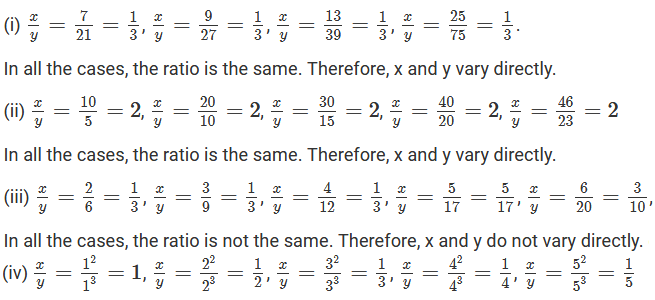Question: 4

Fill in the blanks in each of the following so as to make the statement true:

(i) Two quantities are said to vary… with each other if they increase (decrease) together in such a way that the ratio of the corresponding values remains same.

(ii) x and y are said to vary directly with each other if for some positive number k, …. = k

(iii) If u = 3v, then u and v vary … with each other.

Solution:

(i) Directly

(ii) x and y are said to vary directly with each other if x/y = k, where k is a positive number

(iii) Because u = 3v, u and y vary directly with each other

Question: 5

Complete the following tables given that x varies directly as y.

(i)

x
2.5
—-
—-
15

y
5
8
12
—-

(ii)

x
5
—-
10
35
25
—-

y
8
12
—-
—-
—-
32

(iii)

x
6
8
10
—-
20

y
15
20
—-
40
—-

(iv)

x
4
9
—-
—-
3
—-

y
16
—-
48
36
—-
4

(v)

x
3
5
7
9

y
—-
20
28
—-

Solution:

Here, x and y vary directly. ∴ x = ky

(i) x = 2.5 and y = 5 i.e. 2.5 = 5k

=> 2.5/ 5 = 0.5.

For y = 8 and k = 0.5, we have: x = ky

=> x= 8 X 0.5 = 4

For y = 12 and k = 0.5, we have: x = ky

=> x = 12 X 0.5 = 6

For x = 15 and k = 0.5, we have: x = ky

=> 15 = 0.5 X y

=> y = 15/0.5 = 30

(ii) x = 5 and y = 8 i.e. 5 = 8 X k

=> k = 5/8 = 0.625

For y = 12 and k = 0.625, we have; x = ky

=> x = 12 X 0.625 = 7.5

For x = 10 and k = 0.625, we have: x = ky

=> 10 = 0.625 X y

=> y = 10/0.625 =16

For x = 35 and k = 0.625, we have: x = ky

=> 35 = 0.625 X y

=> y = 35/0.625 = 56

For x = 25 and k = 0.625, we know: x = ky

=> 25 = 0.625 X y

=> y = 25/0.625 = 40

For y = 32 and k = 0.625, we know: x = ky

=> x = 0.625 X 32 = 20

(iii) x = 6 and y = 15 i.e. 6 = 15k

=> k = 6/15 = 0.4

For x = 10 and k = 0.4, we have:

y = 10/0.4 = 25

For y = 40 and k = 0.4, we have:

x = 0.4 X 40 = 16

For x = 20 and k = 0.4, we have: y = 20/0.4 = 50

(iv) x = 4 and y = 16 i.e. 4 = 16k

=> k = 4/16 =1/4

For x = 9 and k = 1/4, we have: 9 = ky

=> y = 4 X 9 = 36

For y = 48 and k = 1/4, we have: x = ky

=> 1/4 × 48 = 12

For y = 36 and k = 14, we have: x = ky

=> 1/4 ×36 = 9

For x = 3 and k = 14, we have: x = ky

=> 3 = 1/4 × y

=> y = 12

For y = 4 and k = 1/4, we have: x = ky

= 1/4 × 4 = 1

(v) x = 5 and y = 20 i.e. 5 = 20k

=> k = 5/20 = 1/4

For x = 3 and k = 1/4, we have:

3 = 1/4 × y

=> y = 12

For x = 9, k = 1/4, we have: x = ky

=> 9 = 1/4 × y

=> y = 36

Question: 6

Find the constant of variation from the table given below:

x
3
5
7
9

y
12
20
28
36

Set up a table and solve the following problems. Use unitary method to verify the answer.

Solution:

Since it is a direct variation, x/y = k.

For x = 3 and y = 12, we have: k = 3/12 = 1/4

Thus, in all cases, k = ¼

Question: 7

Rohit bought 12 registers for Rs 156; find the cost of 7 such registers.

Solution:

Let the cost of y registers be Rs x.

Register
12
7

Cost (in Rs)
156
x

If he buys less number of registers, the cost will also be less. Therefore, it is a direct variation. We get: 12: 7 = 156: x => 12/7 = 156x

Applying cross multiplication, we get: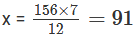Thus, the cost of 7 such registers will be Rs 91.

Question: 8

Anupama takes 125 minutes in walking a distance of 100 meters. What distance would she cover in 315 minutes?

Solution:

Let the distance travelled is more, the time needed to cover it will be more. Therefore, it is direct variation. We get: 125: 135 = 100: xApplying cross multiplication, we get: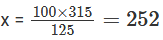Thus, Anupama would cover 252 meter in 315 minutes.

Question: 9

If the cost of 93 m of a certain kind of plastic sheet is Rs 1395, then what would it cost to buy 105 m of such plastic sheet?

Solution:

Length of plastic sheet (in meter)
93
105

Cost (in Rs)
1395
x

Let the cost of the plastic sheet per meter be Rs x

If more sheets are brought, the cost will also be more. Therefore, it is a direct variation. We get: 93: 105 = 1395: x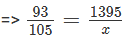Applying cross multiplication, we get: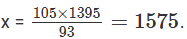Thus, the required cost will be Rs 1,575.

Question: 10

Suneeta types 1080 words in one hour. What is her GWAM (gross words a minute rate)?

Number of words
1080
x

Time (in minute)
60
1

Solution:

Let x be her GWAM.

If the time taken is less, GWAM will also be less. Therefore, it is a direct = 60: 1Applying cross multiplication, we get: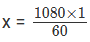= GWAM will be 18.

Question: 11

A car is travelling at the average speed of 50 km/hr. How much distance would it travel in 12 minutes?

Distance (in km)
50
x

Time (in minute)
60
12

Solution:

Let the distance be x km.

If the time taken is less, the distance covered will also be less. Therefore, it is a direct variation. 50: x = 60: 12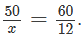Applying cross multiplication, we get: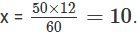Thus, the required distance will be 10 km.

Question: 12

68 boxes of a certain commodity require a shelf- length of 13.6 m. How many boxes of the same commodity would occupy a shelf- length of 20.4 m?

Number of boxes
68
x

Shelf- length (in m)
13.6
20.4

Solution:

Let x be the number of boxes that occupy a shelf- length of 20.4 m

If the length of the shelf increases, the number of boxes will also increase. Therefore, it a case of direct variation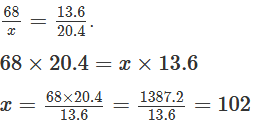Thus, 102 boxes will occupy a shelf- length of 20.4 m.

Question: 13

In a library 136 copies of a certain book required a shelf- length of 3.4 meter. How many copies of the same book would occupy a shelf- length og 5.1 meters?

Number of copies
136
x

Shelf- length (in m)
3.4
5.1

Solution:

Let x be the number of copies that would occupy a shelf- length of 5.1 m

Since the number of copies and the length of the shelf are in direct variation, we have: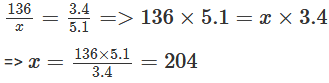Thus, 204 copies will occupy a shelf of length 5.1 m

Question: 14

The second class railway fare for 240 km of journey is Rs 15.00. What would be the fare for a journey of 139.2 km?

Solution:

Let Rs x be the fare for a journey of 139.2 km

Distance (in km)
240
139.2

Fare (in Rs)
15
x

Since the distance travelled and the fares are in direct variation, we have: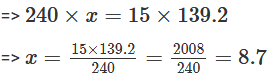Thus, the fare for a journey of 139.2 km will be Rs 8.70

Question: 16

If the thickness of a pile of 12 cardboard is 35 mm, find the thickness of a pile of 294 cardboard.

Solution:

Let x cm be the thickness of a pile of 294 cardboard

Thickness (in cm)
3.5
x

Cardboard
12
294

Since the pile of the cardboard and its thickness are in direct variation, we have:Thus, the thickness of a pile of 294 cardboard will be 85.75 cm (or 857.5 mm).

Question: 16

The cost of 97 meter of cloth is Rs 242.50. What length of this can be purchased for Rs. 302.50?

Solution:

Let x meter be the length of the cloth that can be purchased for Rs. 320.50

Since the length of the cloth and its cost are in direct variation, we have: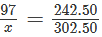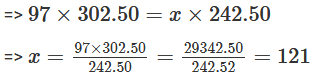Thus, the required length will be 121 meter.

Question: 17

11 men can dig 6(3/4) meter long trench in one day. How many men should be employed for digging 27 meter long trench of the same type in one day?

Solution:

Let x be the number of men required to dig a trench of 27 meter.

Number of men
11
x

Length (in m)
27/4
27

Since the length of the trench and the number of men are in direct variation, we have:Thus, 44 men will be required to dig a trench of 27 meter.

Question: 18

A worker is paid Rs 210 for 6 days work. If his total income of the month is Rs 875, for how many days did he work?

Solution:

Let x be the number of days for which the worker is paid Rs 875.

Income (in Rs)
210
875

Number of days
6
x

Since the income of the worker and the number of working days are in direct variation, we have: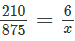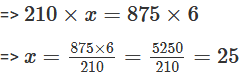Thus, the required number of days is 25.

Question: 19

A worker is paid Rs 200 for 8 days work. If he works for 20 days, how much will he get?

Solution:

Let Rx x be the income for 20 days work

Income (in Rs)
200
x

Number of days
8
20

Since the income and the number of working days are in direct variation, we have:Thus, the worker will get Rs 500 for working 20 days.

Question: 20

The amount of extension in an elastic string varies directly as the weight hung on it. If a weight of 150 gm produces an extension of 2.9 cm, then what weight would produce an extension of 17.4 cm?

Solution:

Let x gm be the weight that would produce an extension of 17.4 cm.

Weight (in gm)
150
x

Length (in cm)
2.9
17.4

Since the amount of extension in an elastic string and the weight hung on it are in direct variation, we have: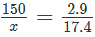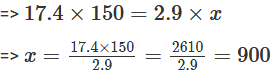Thus, the required weight will be 900 gm.

Question: 21

The amount of extension in an elastic spring varies directly with the weight hung on it. If a weight of 250 gm produces an extension of 3.5 cm, find the extension produced by a weight of 700 gm.

Solution:

Let x cm be the extension produced by the weight of 700 gm

Weight (in gm)
250
700

Length (in cm)
3.5
x

Since the amount of extension in an elastic spring varies and the weight hung on it is in direct variation, we have: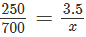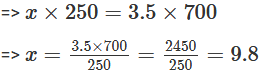Thus, the required extension will be 9.8 cm

Question: 22

In 10 days, the earth picks up 2.6 × 108 pounds of dust from the atmosphere. How much dust will it pick up in 45 days?

Solution:

Let the amount of dust picked up by the earth in 45 days be x pounds.

Since the amount of dust picked up by the earth and the number of days are in direct variation, we have: Ratio of the dust picked up by the earth in pounds = ratio of the number of days taken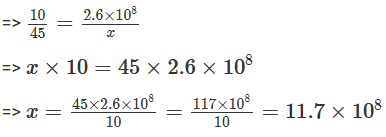Thus, 11.7 × 108 pounds of dust will be picked up by the earth in 45 days.

Question: 23

In 15 days, the earth picks up 1.2 × 108 kg of dust from the atmosphere. In how many days it will pick up 4.8 × 108 kg of dust?

Solution:

Let x be the number of days taken by the earth to pick up 4.8 × 108 kg of dust

Since the amount of dust picked up by the earth and the number of days are in direct variation, we get:Thus, the required number of days will be 60.

```### Course Features

• 728 Video Lectures
• Revision Notes
• Previous Year Papers
• Mind Map
• Study Planner
• NCERT Solutions
• Discussion Forum
• Test paper with Video Solution